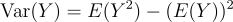09 Oct 2023

Posted on:

09 Oct 2023

0

# Resolved:using the Expected Value to estimate the variance of the Logistic Distribution?

at 3:29, the instructor mentions you can use the Expected Value to estimate the Variance of the Logistic Distribution.

However, in the Variance Formula it makes no reference/does not use the Expected Value as an input? so how is the Expected Value used to estimate the variance of the Logistic Distribution?

thanks

Instructor
Posted on:

09 Oct 2023

1

Hey Ryan,

Thanks for reaching out!

The variance of a random variable can be calculated from its expectation value using the following expression:Following this formula, the derivation of the variance of a logistic distribution is more invloved than the derivation of others and the lecturer has decided to only show the end result. Still, you are welcome to study the proof using, for example, the Proof Wiki page:

https://proofwiki.org/wiki/Variance_of_Logistic_Distribution/Proof_1

Hope this helps.

Kind regards,

365 Hristina